Courses

# Test: Building With Bricks - 3

## 10 Questions MCQ Test Mathematics (Maths) for Class 4 | Test: Building With Bricks - 3

Description
This mock test of Test: Building With Bricks - 3 for Class 4 helps you for every Class 4 entrance exam. This contains 10 Multiple Choice Questions for Class 4 Test: Building With Bricks - 3 (mcq) to study with solutions a complete question bank. The solved questions answers in this Test: Building With Bricks - 3 quiz give you a good mix of easy questions and tough questions. Class 4 students definitely take this Test: Building With Bricks - 3 exercise for a better result in the exam. You can find other Test: Building With Bricks - 3 extra questions, long questions & short questions for Class 4 on EduRev as well by searching above.
QUESTION: 1

### The given figure contains ________ triangles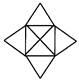Solution:

The triangles formed are.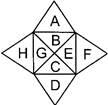A, B, C, D, E, F., G, H, BG, BE, CE and CG, i.e., 12 in number

QUESTION: 2

### Which of the following dotted lines represents the line of symmetry?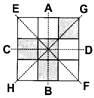Solution:

GH symmetrically divides the figure.

QUESTION: 3

###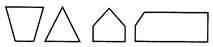are all __.

Solution:

All are closed figures with different number of sides and a closed figure is called a polygon.

QUESTION: 4

What is the perimeter of the given figure?Solution:

Perimeter of the given figure = 44 cm.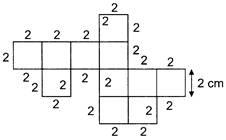QUESTION: 5

In the given diagram, what is the least number of squares that must be shaded so that the given figure is symmetrical along the dotted line?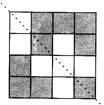Solution:

Only one square must be shaded to divide the figure into two equal parts.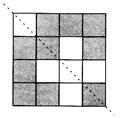QUESTION: 6

How many squares are there in the given figure?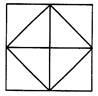Solution: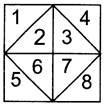The squares formed are 12, 34, 56, 78, 2367 and 12345678 i.e., 6 in number.

QUESTION: 7

Find the length of GH.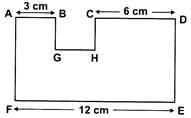Solution:

We have,
AB + GH + CD = FE
⇒ 3 cm + GH + 6 cm = 12 cm
⇒ GH = 12 cm - 9 cm = 3 cm

QUESTION: 8

Which of the following figures has the highest perimeter?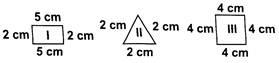Solution:

Perimeter of a figure is the sum of all of its sides.
Perimeter of I=5+2+5+2= 14cm Perimeter of II = 2+2+2=6cm
Perimeter of III = 4+4+4+4=16cm Hence III has highest perimeter,

QUESTION: 9

Which line in the diagram is the correct line of symmetry?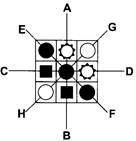Solution:

GH symmetrically divides the figure.

QUESTION: 10

IJKL and MNOP are two rectangles. Find the length of KP.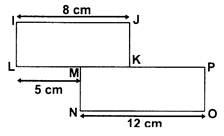Solution:

In rectangle IJKL, we have
IJ = LM + MK (opposite sides are equal in a rectangle.) ⇒8=5+MK
∴ MK = 3 cm
In rectangle MNOP, we have
MP = NO ⇒ MK + KP = NO
⇒ 3 + KP = 12
⇒ KP = 9 cm.